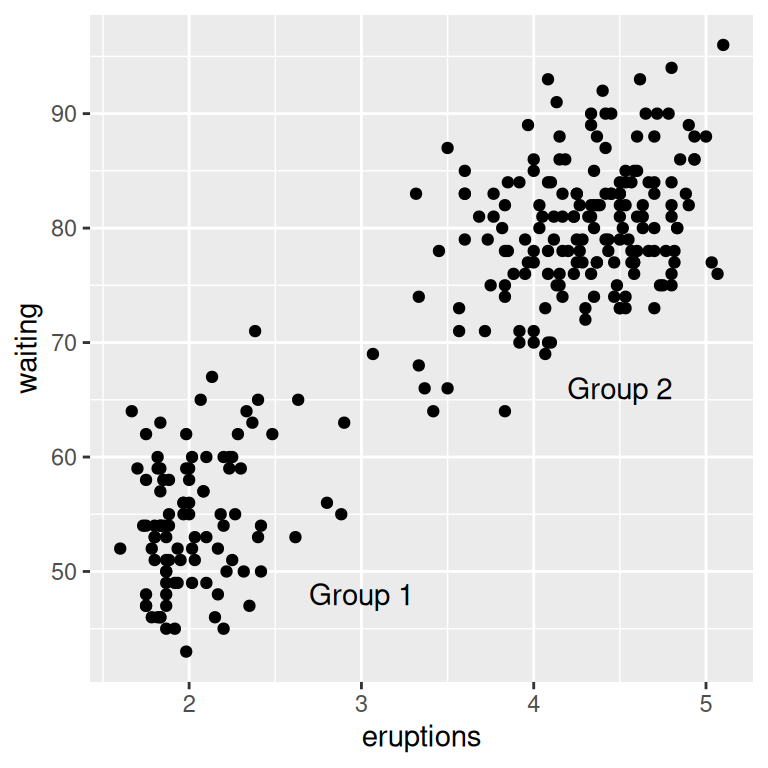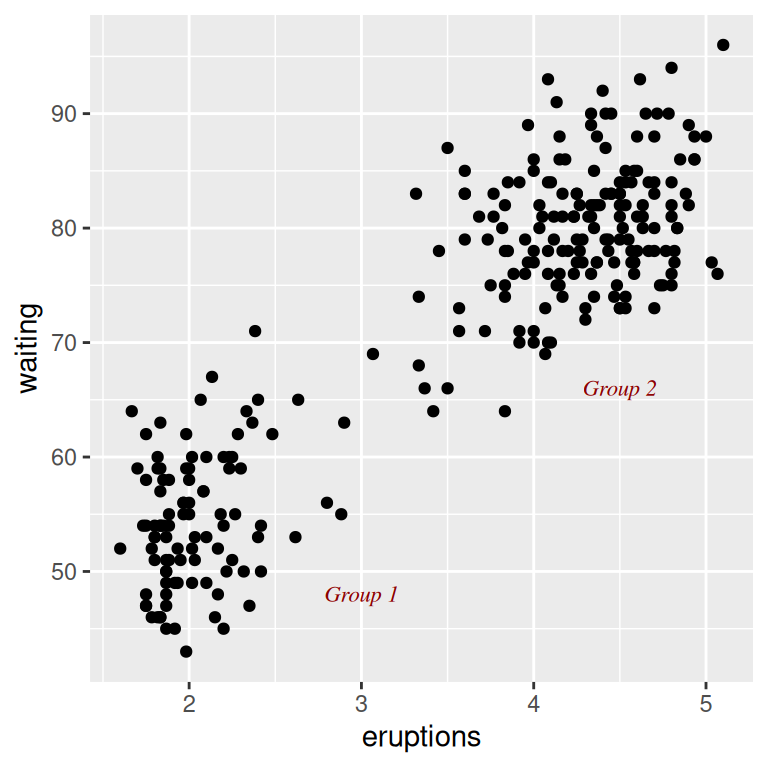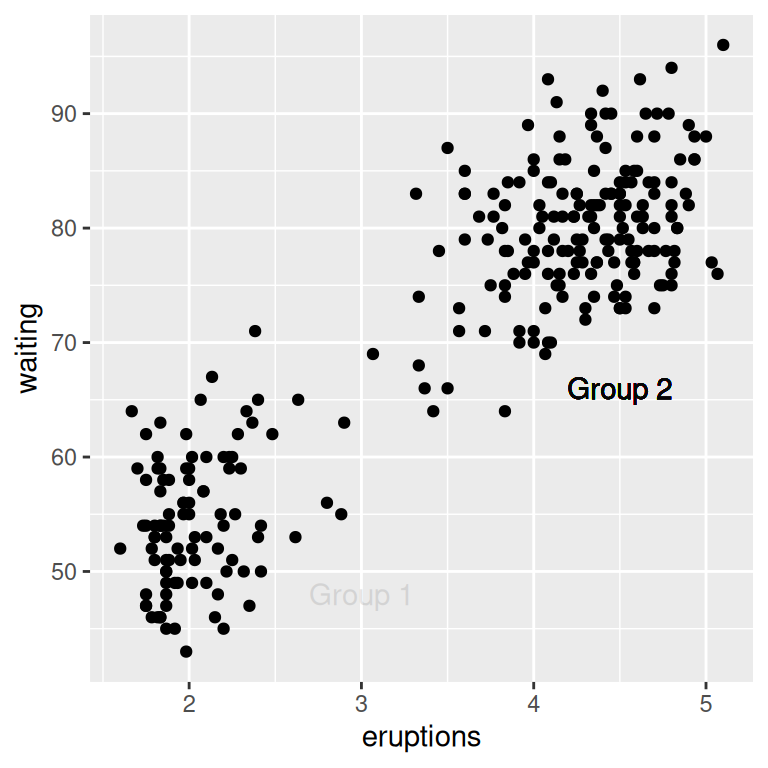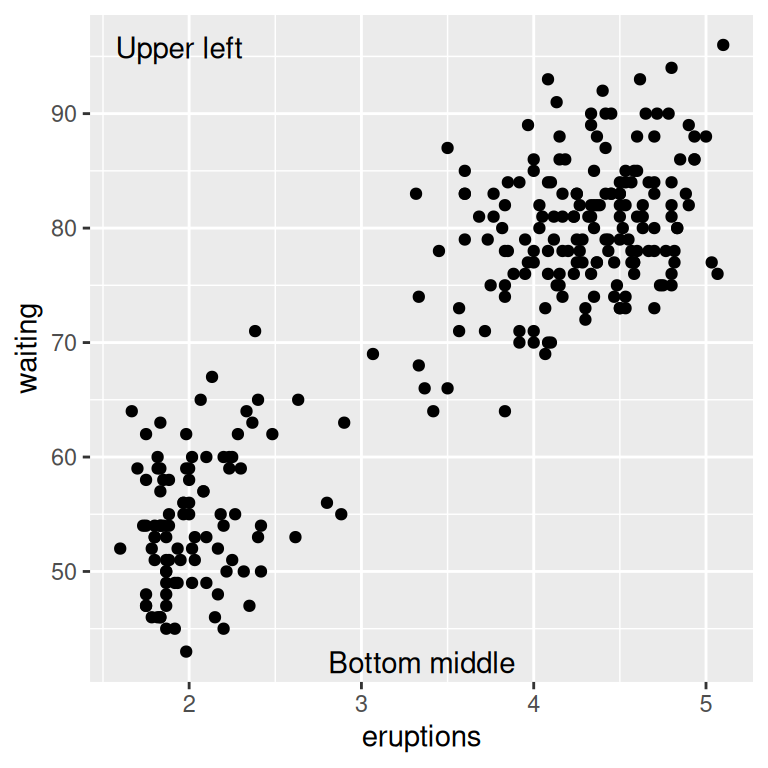### 7.1.1 Problem

You want to add a text annotation to a plot.

### 7.1.2 Solution

Use `annotate()` and a text geom (Figure 7.1):

``````p <- ggplot(faithful, aes(x = eruptions, y = waiting)) +
geom_point()

p +
annotate("text", x = 3, y = 48, label = "Group 1") +
annotate("text", x = 4.5, y = 66, label = "Group 2")``````Figure 7.1: Text annotations

### 7.1.3 Discussion

The `annotate()` function can be used to add any type of geometric object. In this case, we used `geom = "text"`.

Other text properties can be specified, as shown in Figure 7.2:

``````p +
annotate("text", x = 3, y = 48, label = "Group 1",
family = "serif", fontface = "italic", colour = "darkred", size = 3) +
annotate("text", x = 4.5, y = 66, label = "Group 2",
family = "serif", fontface = "italic", colour = "darkred", size = 3)``````Figure 7.2: Modified text properties

Be careful not to use `geom_text()` when you want to add individual text objects. While `annotate(geom = "text")` will add a single text object to the plot, `geom_text()` will create many text objects based on the data, as discussed in Recipe 5.11.

If you use `geom_text()`, the text will be heavily overplotted on the same location, with one copy per data point:

``````p +
# Normal
annotate("text", x = 3, y = 48, label = "Group 1", alpha = .1) +
# Overplotted
geom_text(x = 4.5, y = 66, label = "Group 2", alpha = .1)``````Figure 7.3: Overplotting one of the labels – both should be 90% transparent

In Figure 7.3, each text label is 90% transparent, making it clear which one is overplotted. The overplotting can lead to output with aliased (jagged) edges when outputting to a bitmap.

If the axes are continuous, you can use the special values `Inf` and `-Inf` to place text annotations at the edge of the plotting area, as shown in Figure 7.4. You will also need to adjust the position of the text relative to the corner using `hjust` and `vjust` – if you leave them at their default values, the text will be centered on the edge. It may take a little experimentation with these values to get the text positioned to your liking:

``````p +
annotate("text", x = -Inf, y = Inf, label = "Upper left", hjust = -.2, vjust = 2) +
annotate("text", x = mean(range(faithful\$eruptions)), y = -Inf, vjust = -0.4,
label = "Bottom middle")``````Figure 7.4: Text positioned at the edge of the plotting area